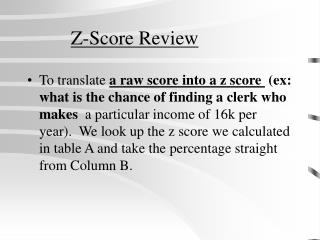DownloadDownload PresentationZ-Score Review

# Z-Score Review

Télécharger la présentation## Z-Score Review

- - - - - - - - - - - - - - - - - - - - - - - - - - - E N D - - - - - - - - - - - - - - - - - - - - - - - - - - -
##### Presentation Transcript

1. Z-Score Review • To translate a raw score into a z score (ex: what is the chance of finding a clerk who makes a particular income of 16k per year). We look up the z score we calculated in table A and take the percentage straight from Column B.

2. If we are asked to find what percentage of a distribution lies at or beyond a particular raw score: • For example a raw score of 16k • we calculate the z score, look that up in table A and take the percentage from column C of table A as our answer (whether the z score is negative or positive).

3. Z scores and percentile rank • We use z scores in conjunction with standardized testing scores to figure at how the score we got measures up. • If our calculated z score is above the mean (positive z score), we look up our z score in table A, column B. Since we are above the mean we have to add 50% to whatever the figure is in column B. • For example if column B gave us an answer of 22.57%, we would add 50% to that because we need to account for the other half of the scores that are below the mean. Which would tell us that our percentile rank is 72.57 or that about 72% of the people who took the test scored worse than we did.

4. If our calculated z score is below the mean (a negative z score) and we are trying to find our percentile rank, we look up the z score in Table A, column C and use that direct percentage. • We don’t add 50% because we are already below the mean.

5. To find the percentile that falls between two different z scores: • First we calculate the z score for each one. For example if we are looking for the percentile of scores between 88 and 120: Z = 120.0-100.0 = 1.25 16.0 Z = 88.0-100.0 = -0.75 16.0 • Then we find the area for both(Table A, column B). For the area between the mean and z of 1.25 it is 39.44%. For the area between –0.75 and the mean is 27.34%. We add these together and get 39.44% + 27.34% = 66.78%. That tells us that 66.78 percent of scores fall between the scores of 88 and 120.